# NCERT Solutions for Class 12 Maths Chapter 5 Continuity and Differentiability Miscellaneous Exercise

These NCERT Solutions for Class 12 Maths Chapter 5 Continuity and Differentiability Miscellaneous Exercise Questions and Answers are prepared by our highly skilled subject experts.

## NCERT Solutions for Class 12 Maths Chapter 5 Continuity and Differentiability Miscellaneous ExerciseQuestion 1.
(3x² -9x + 5)9
Solution:
Let y = (3x² -9x + 5)9
∴ $$\frac { dy }{ dx }$$ = (3x² -9x + 5)8. $$\frac { d }{ dx }$$(3x² -9x + 5)
= 9(3x² -9x + 5)8 (6x – 9)
= 27 (3x² -9x + 5)8 (2x – 3)

Question 2.
sin³x + cos6 x
SoL
Let y = sin³x + cos6 x
∴ $$\frac { dy }{ dx }$$ = 3 sin²x . cosx + 6cos5x (- sinx)
= 3 sinx cosx (sinx – 2 cos4x)Question 3.
(5x)3cos2x
Solution:
Let y = (5x)3cos2x
Taking logarithmon both sides,
∴ log y = 3 cos 2x log 5x
Differentiating both sides, w.r.t. x,Question 4.
sin-1 (x$$\sqrt{x}$$), 0 ≤ x ≤ 1
Solution:Question 5.
$$\frac{\cos ^{-1} \frac{x}{2}}{\sqrt{2 x+7}},-2<x<2$$
Solution: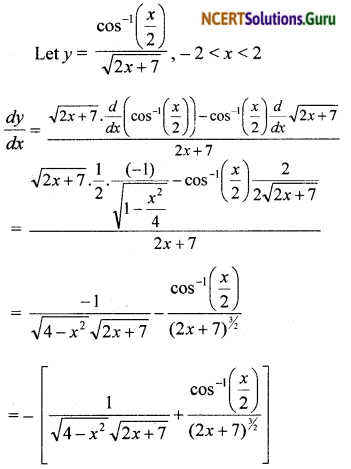Question 6.
$$\cot ^{-1}\left[\frac{\sqrt{1+\sin x}+\sqrt{1-\sin x}}{\sqrt{1+\sin x}-\sqrt{1-\sin x}}\right], 0<x<\frac{\pi}{2}$$
Solution:Question 7. $$(\log x)^{\log x}, x>1$$
Solution:
Let y = $$(\log x)^{\log x}$$
Taking logarithmon both sides,
∴ log y = log x(log log x)
Differentiating both sides, w.r.t. x,
$$\frac { 1 }{ y }$$ $$\frac { dy }{ dx }$$ = logx.$$\frac { 1 }{ log x }$$.$$\frac { 1 }{ x }$$ + $$\frac { 1 }{ x }$$.log log x
∴ $$\frac { dy }{ dx }$$ = (log x)log x [$$\frac { 1 }{ x }$$ + $$\frac { log log.x }{ x }$$]Question 8.
cos (a cos x + b sin x), for sorne constant a and b.
Solution:
Let y = cos (a cosx + b sinx)
$$\frac { dy }{ dx }$$ = sin (a cosx + h sinx).
$$\frac { dy }{ dx }$$(a cosx + b sinx)
= – sin (a cosx + b sinx) [- a sinx + b cosx]
= (a sinx – b cosx).sin (a cosx + b sinx)

Question 9.
(sin x – cos x)sin x-cos x, $$\frac { π }{ 4 }$$< x < $$\frac { 3π }{ 4 }$$
Solution:
When $$\frac { π }{ 4 }$$< x < $$\frac { 3π }{ 4 }$$, then sin x > cos x
so that sin x – cos x is positive.
Let y = (sinx – cosx)sin x-cos x
Taking logarithm on both sides,
∴ logy = (sinx – cosx) log (sinx – cosx)
Differentiating both sides w.r.t. x,
$$\frac { 1 }{ y }$$ $$\frac { dy }{ dx }$$
=( sinx – cosx)$$\frac { 1 }{ sin x-cosx }$$ .$$\frac { d }{ dx }$$(sinx-cosx) + log (sinx – cosx). (cosx + sinx)
= (cosx + sinx) + log (sinx – cosx) . (cosx + sinx)
= (cosx + sinx) [1 + log (sinx – cosx)]
∴ $$\frac { dy }{ dx }$$ = (sin x – cos x)sin x-cos x(cosx + sinx) [1 + log(sinx – cosx)]Question 10.
xx + xa + ax + aa for some fixed a > 0 and x > 0.
Solution: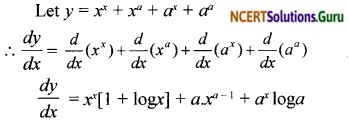Question 11.
$$x^{x^{2}-3}+(x-3)^{x^{2}}$$, for x > 3
Solution:
Let u = x$$x^{x^{2}-3}$$ and v = (x – 3)
Differentiating w.r.t. x,
∴ $$\frac { dy }{ dx }$$ = $$\frac { du }{ dx }$$ + $$\frac { dv }{ dx }$$ … (1)
u = xx²-3
Taking logarithm on bath sides,
∴ log u = (x² – 3)log
Differentiating both sides w.r.t. x,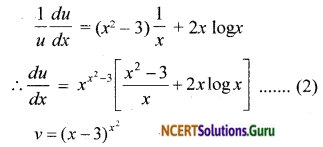Taking logarithm on bath sides,
∴ log v = x²log(x – 3)
Differentiating both sides w.r.t. x,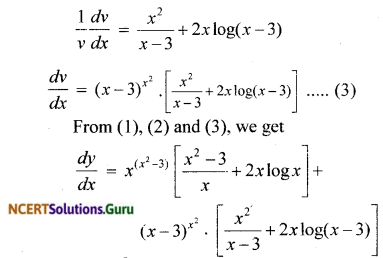Question 12.
Find $$\frac { dy }{ dx }$$, if y = 12(1 – cos t),
x = 10(t – sin t), $$\frac { – π }{ 2 }$$ < t < $$\frac { π }{ 2 }$$
Solution: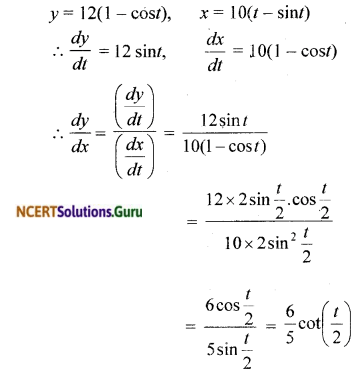Question 13.
Find $$\frac { dy }{ dx }$$, if
y = sin-1 x + sin-1 $$\sqrt{1-x^{2}},-1 \leq x \leq 1$$.
Solution:Question 14.
If $$x \sqrt{1+y}+y \sqrt{1+x}$$ = 0, for – 1 < x < 1, prove that $$\frac { dy }{ dx }$$ = – $$\frac{1}{(1+x)^{2}}$$
Solution:
$$x \sqrt{1+y}+y \sqrt{1+x}$$ = 0
$$x \sqrt{1+y}$$ = – y$$\sqrt{1+y}$$
Squaring both sides,
x²(1 + y) = y²(1 + x)
x² + x²y = y² + y²x
x² – y² = y²x – x²y
(x – y)(x + y) = xy(x – y)
(x + y) = – xy
y + xy = – x
y(1 + x) = – x
∴ y = $$\frac { – x }{ 1+x }$$
Differentiating w.r.t. x,
$$\frac { dy }{ dx }$$ = $$\frac{(1+x)(-1)-(-x) 1}{(1+x)^{2}}$$
= $$\frac{-1-x+x}{(1+x)^{2}}$$ = $$\frac{-1}{(1+x)^{2}}$$, x ≠ – 1Question 15.
If (x – a)² + (y – b)² = c², for some c > 0, prove that
$$\frac{\left[1+\left(\frac{d y}{d x}\right)^{2}\right]^{\frac{3}{2}}}{\frac{d^{2} y}{d x^{2}}}$$
is a constant, independent of a and b.
Solution:Question 16.
If cos y = x cos (a + y), with
cos a ≠ ± 1, prove that $$\frac { dy }{ dx }$$ = $$\frac{\cos ^{2}(a+y)}{\sin a}$$
Solution: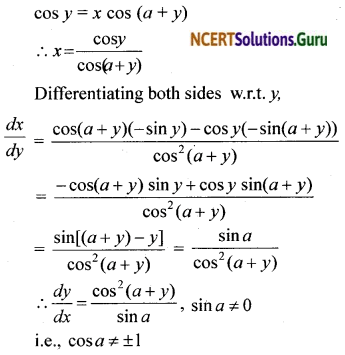Question 17.
If x = a(cos t + t sin t) and y = a(sin t – t cos t), find $$\frac{d^{2} y}{d x^{2}}$$
Solution: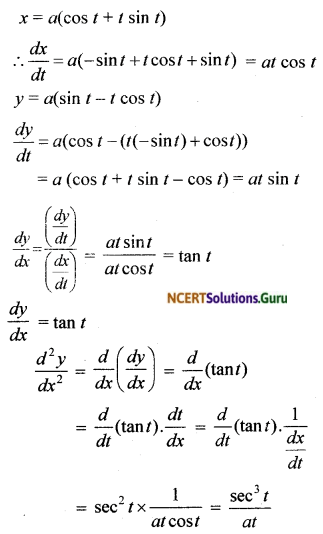Question 18.
If f(x) = |x|³, show that f”(x) exists for all real x and find it.
Solution:
f(x) can be redefined as
f(x) = \left\{\begin{aligned} x^{3}, & x \geq 0 \\ -x^{3}, & x<0 \end{aligned}\right. For x > 0 and x < 0, fix) is a polynomial function. Hence f(x) is differentiable for x > 0 and x < 0. ∴ For x > 0, f'(x) = 3x² and f”(x) = 6x
For x < 0, f'(x) = – 3x² and f”(x) = – 6x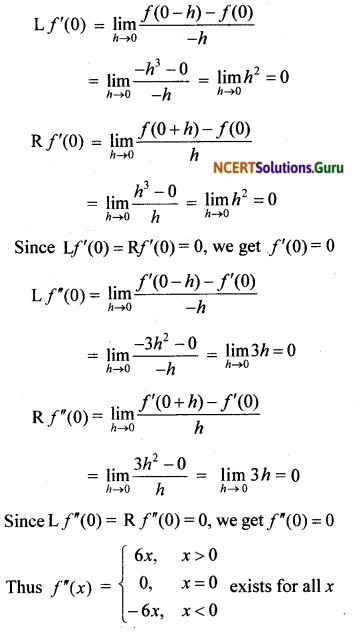or f”(x) = 6|x| exists for all x ∈ R

Question 19.
Using mathematical induction, prove that $$\frac { d }{ dx }$$(xⁿ) = $$n x^{n^{-1}}$$ for all positive integers n.
Solution: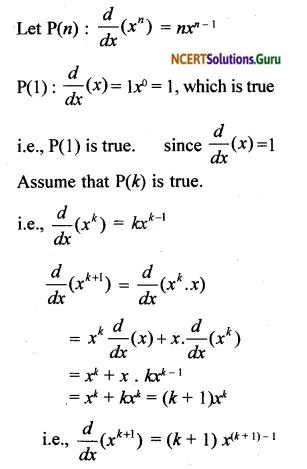Hence P(k + 1) is true.
i.e., P(k + 1) is true whenever P(k) is true.
Hence by the principle of mathematical induction, $$\frac { d }{ dx }$$(xⁿ) = nxn-1 is true for positive integer n.Question 20.
Using the fact that sin (A + B) = sin A cos B + cos A sin B and the differentiation, obtain the sum formula for cosines.
Solution:
sin (A + B) = sin A cos B + cos A sin B
Differentiating both sides w.r.t. x,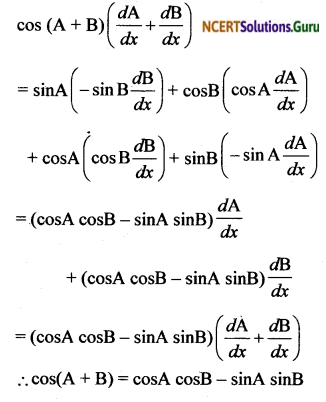Question 21.
Does there exist a function which is continuous everywhere but not differentiable at exactly two points? Justify your answer.
Solution:
Yes.
i. Let f(x) = |x – 1| + |x – 2|
Let g(x) = |x|, h(x) = x – 1, k(x) = x – 2.
Then g(x), h(x) and k(x) are continuous functions since h(x) and k(x) are polynomial functions and g(x) ¡s the modulus function.at x = 1, since Lf’(1) ≠ Rf’(1)
Similarly we can show that f(x) ¡s not differentiable at x = 2.
Thus f(x) = |x – 1| + |x – 2| is continuous everywhere and not differentiable at exactly two points, namely at x = 1 or x = 2.Question 22.
If y = $$\left|\begin{array}{ccc} f(x) & g(x) & h(x) \\ l & m & n \\ a & b & c \end{array}\right|$$, prove that $$\frac { dy }{ dx }$$ = $$\left|\begin{array}{ccc} f^{\prime}(x) & g^{\prime}(x) & h^{\prime}(x) \\ l & m & n \\ a & b & c \end{array}\right|$$
Solution:Question 23.
If y = $$e^{a \cos ^{-1} x}$$, – 1 ≤ x ≤ 1, show that (1 – x²)$$\frac{d^{2} y}{d x^{2}}-x \frac{d y}{d x}-a^{2} y$$ = 0
Solution:
f(x) = x + $$\frac { 1 }{ x }$$
f(x) is a continuous function in [1, 3]
Ax) is differentiable in(1,3)
f’(x) = 1 + $$\\frac{-1}{x^{2}}$$ exists for x ∈ (1, 3)error: Content is protected !!